# Selina Solutions Concise Mathematics Class 6 Chapter 32 Perimeter and Area of Plane Figures Exercise 32(A)

Selina Solutions Concise Mathematics Class 6 Chapter 32 Perimeter and Area of Plane Figures are available in PDF format to boost the exam preparation of students. The method of determining the perimeter of rectangle, square, triangle and circle are the major concepts, discussed under this exercise. Various solved examples are provided to help students understand the method of solving the tricky problems without any difficulty. The solutions, well structured by experts, are available online and offline, to help students in clearing their doubts instantly. Students can avail Selina Solutions Concise Mathematics Class 6 Chapter 32 Perimeter and Area of Plane Figures Exercise 32(A) PDF, from the links provided here.

## Selina Solutions Concise Mathematics Class 6 Chapter 32 Perimeter and Area of Plane Figures Exercise 32(A) Download PDF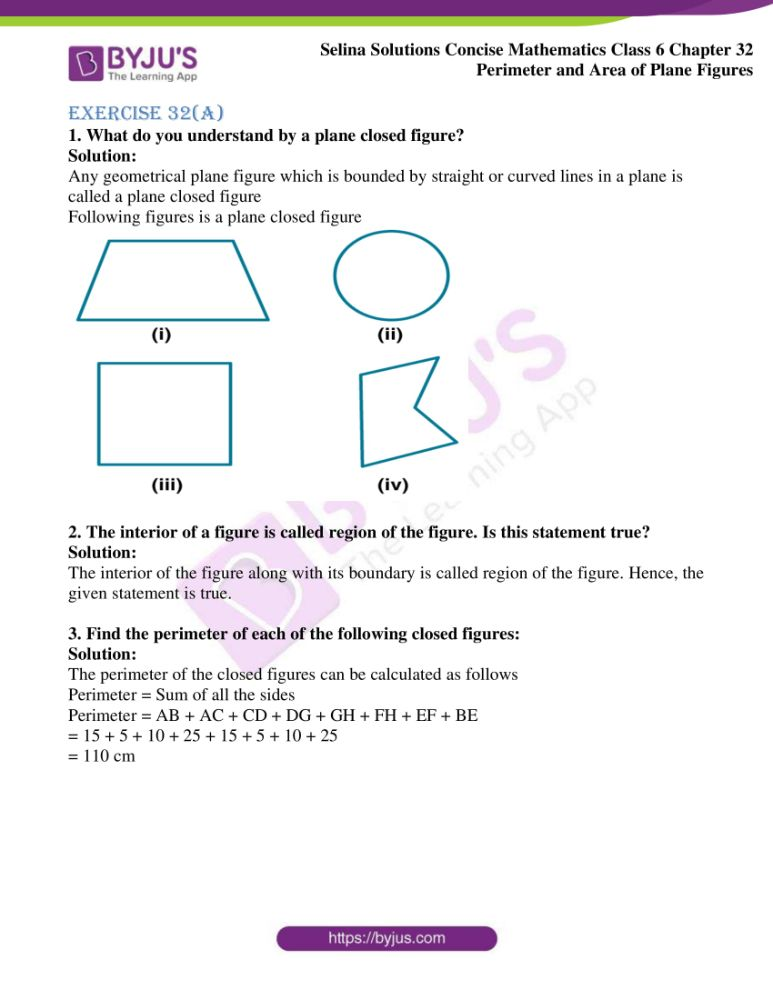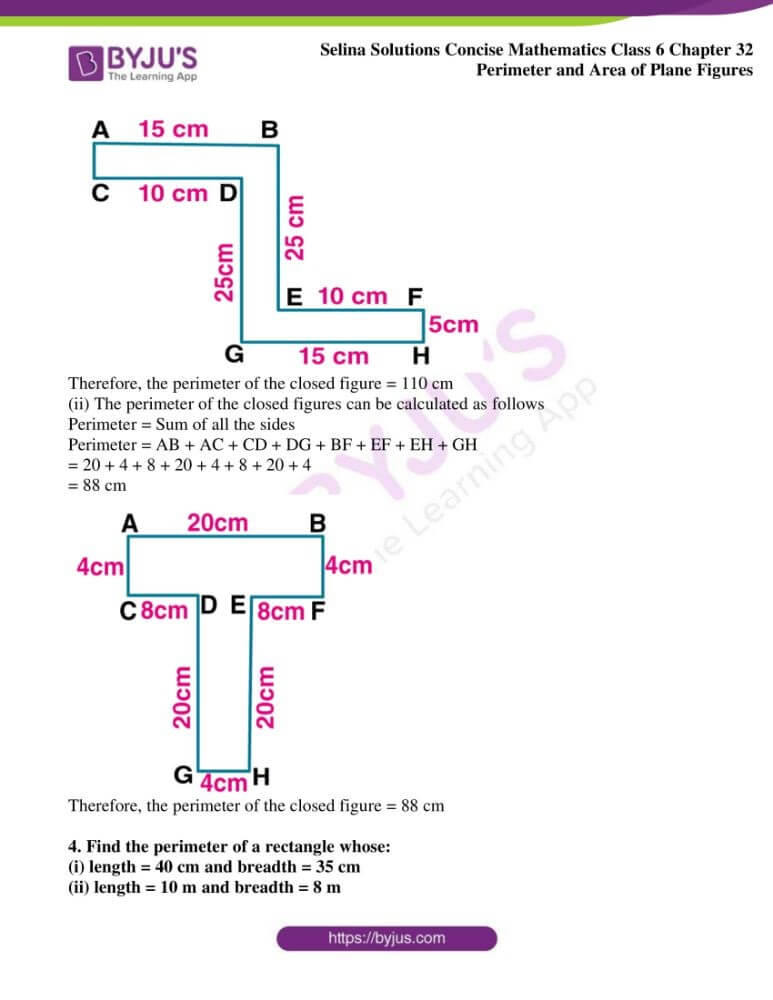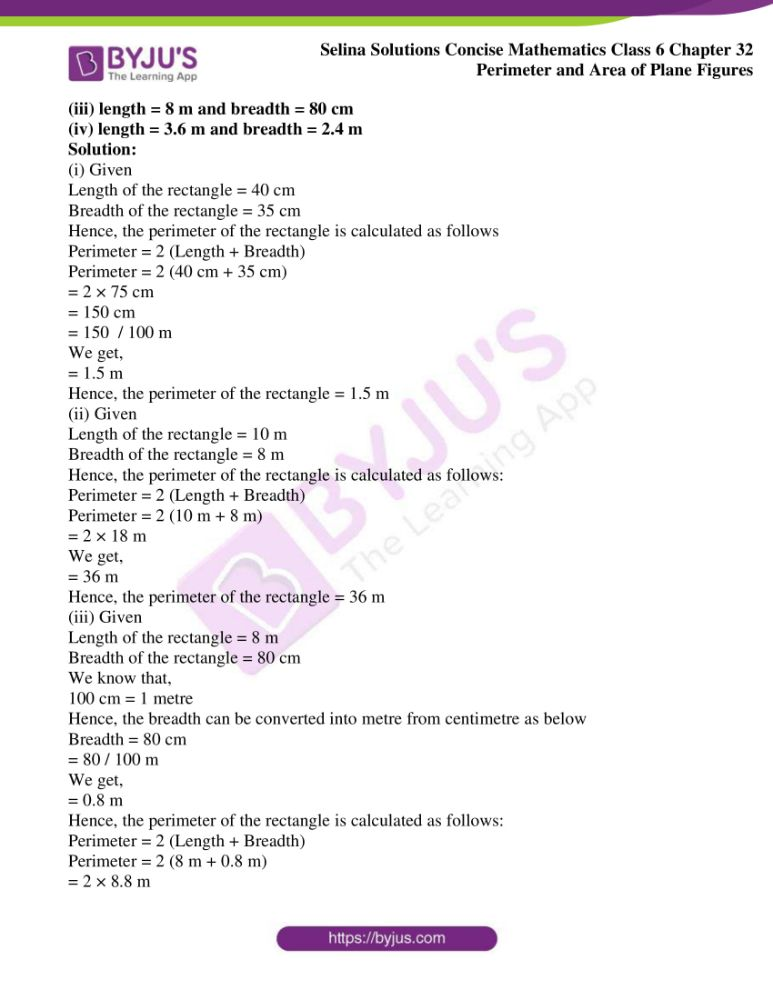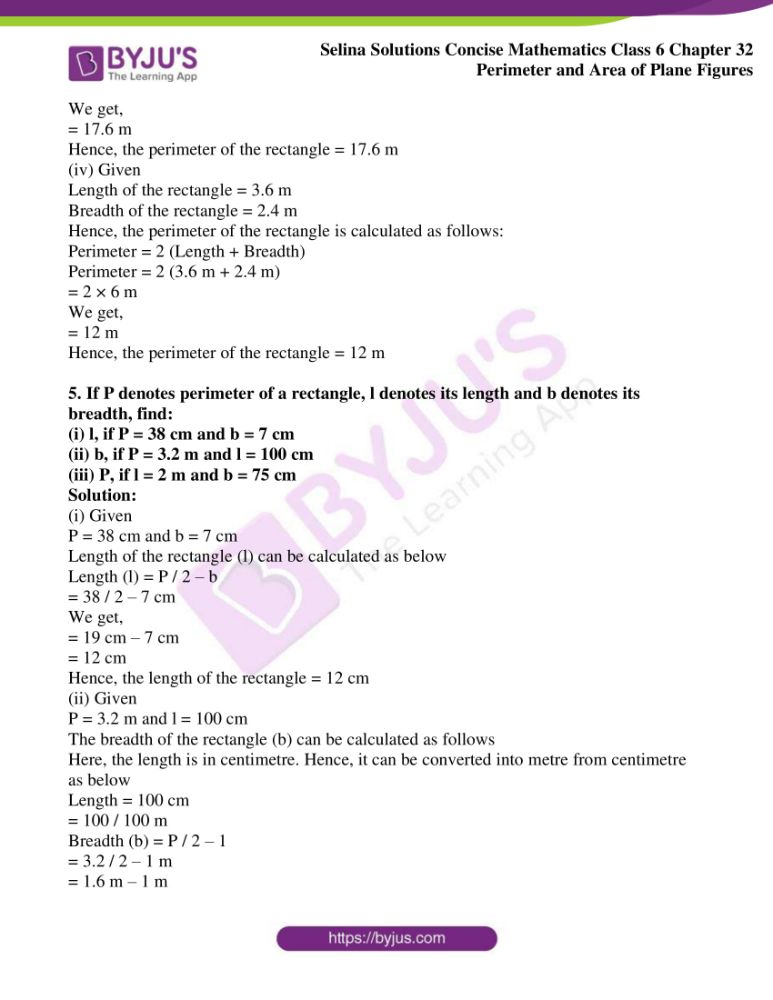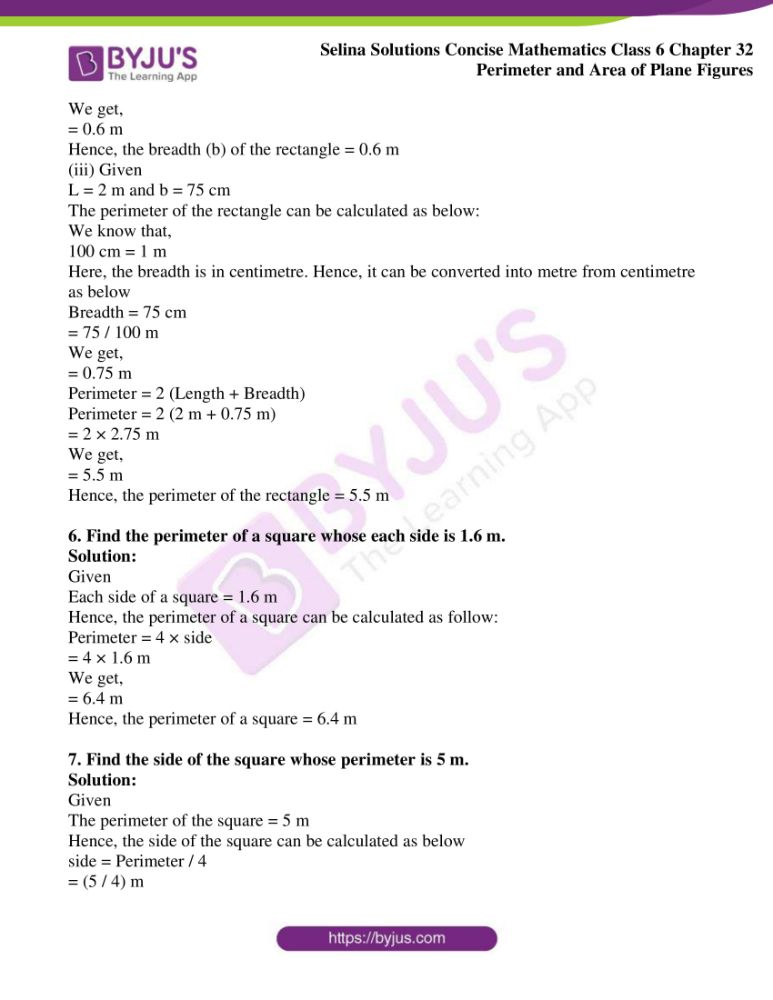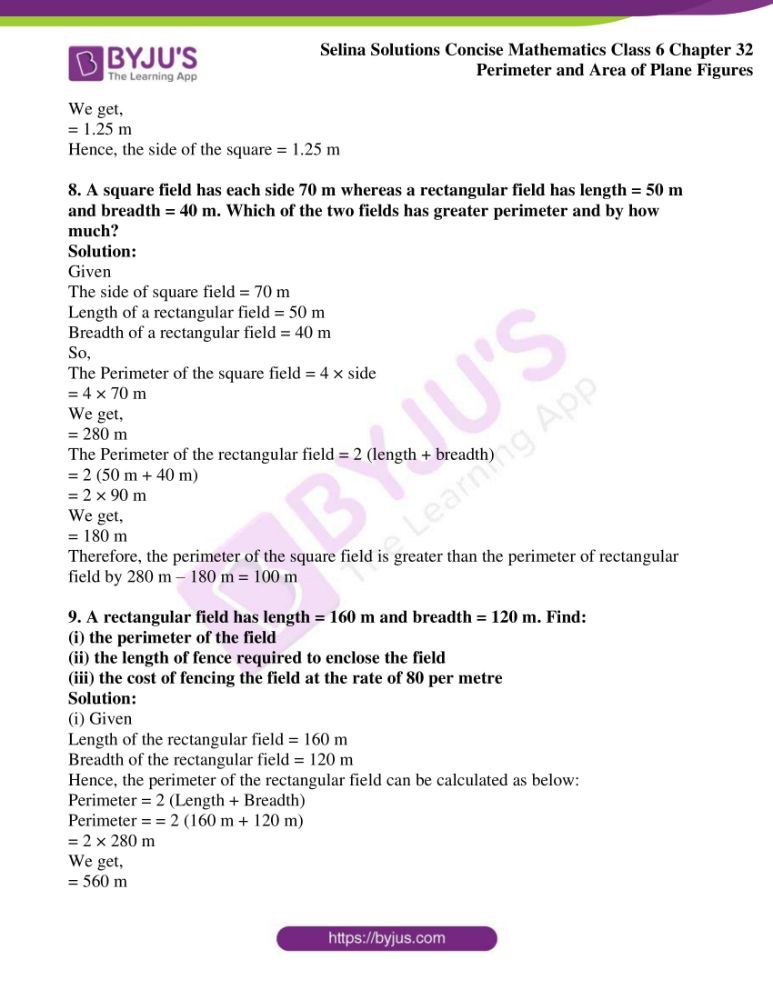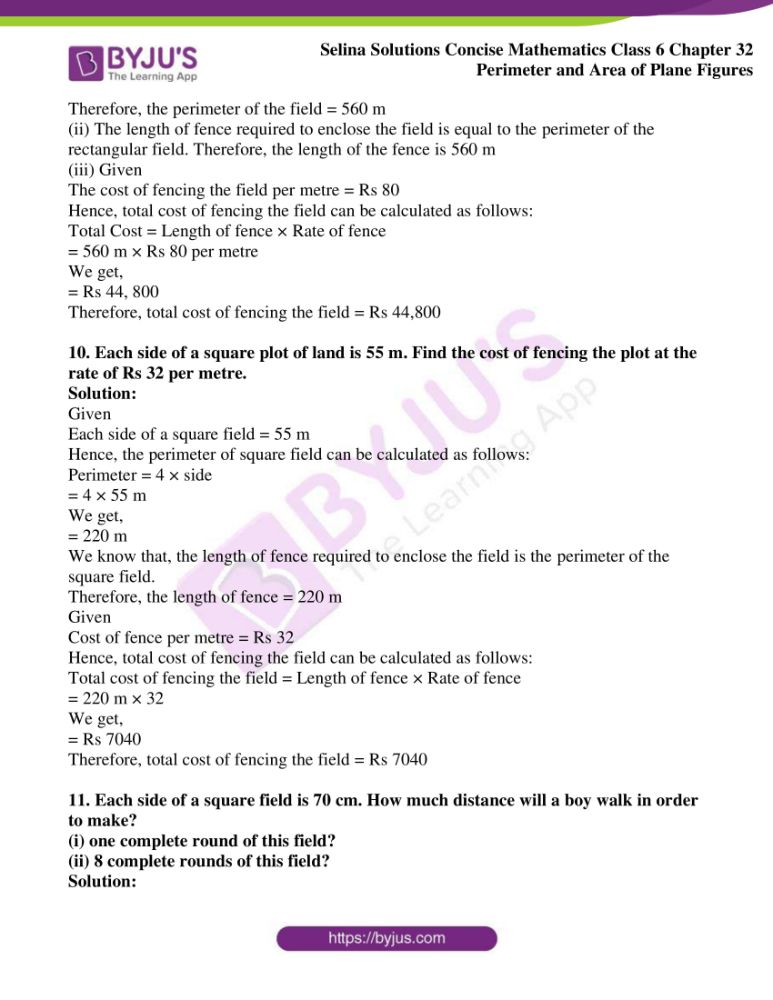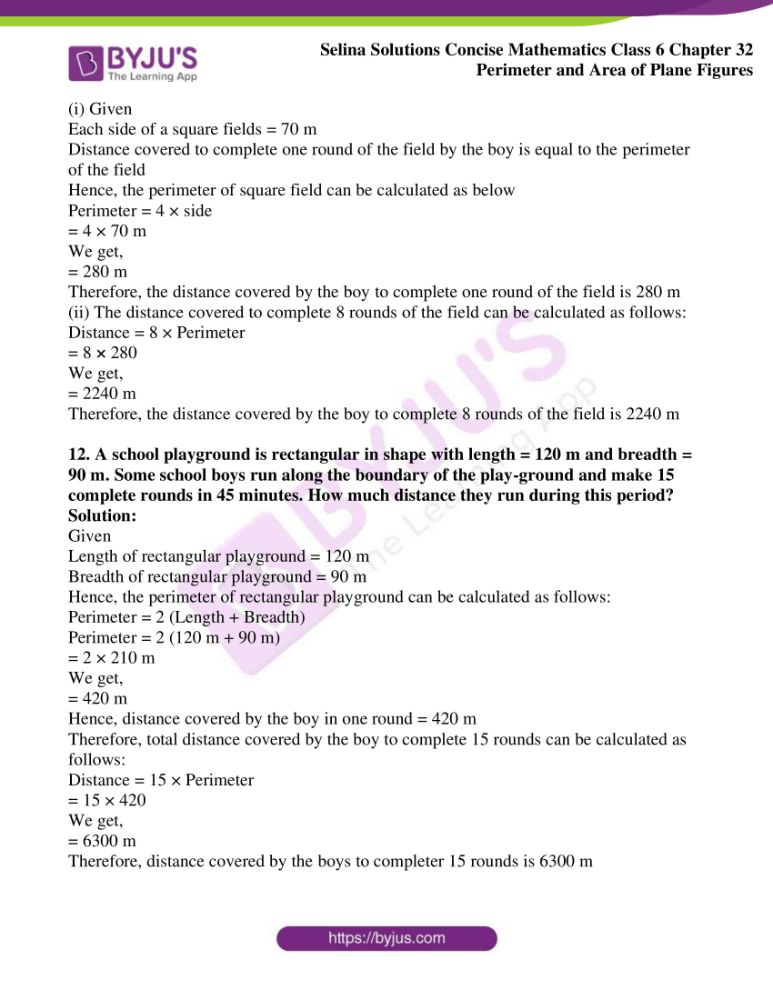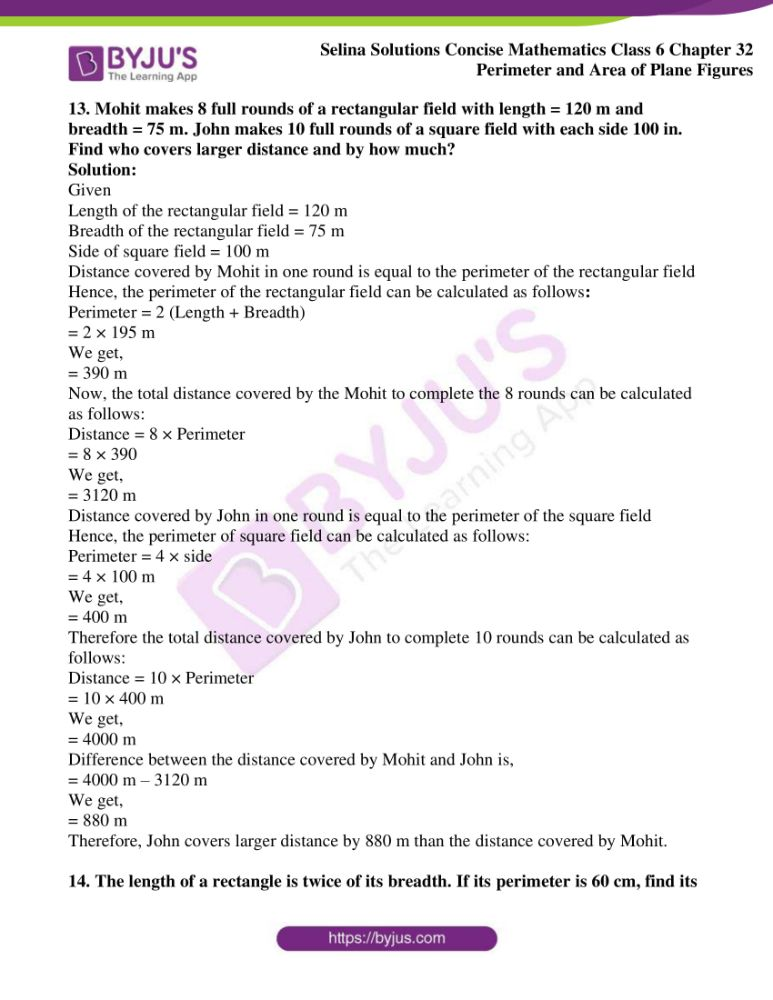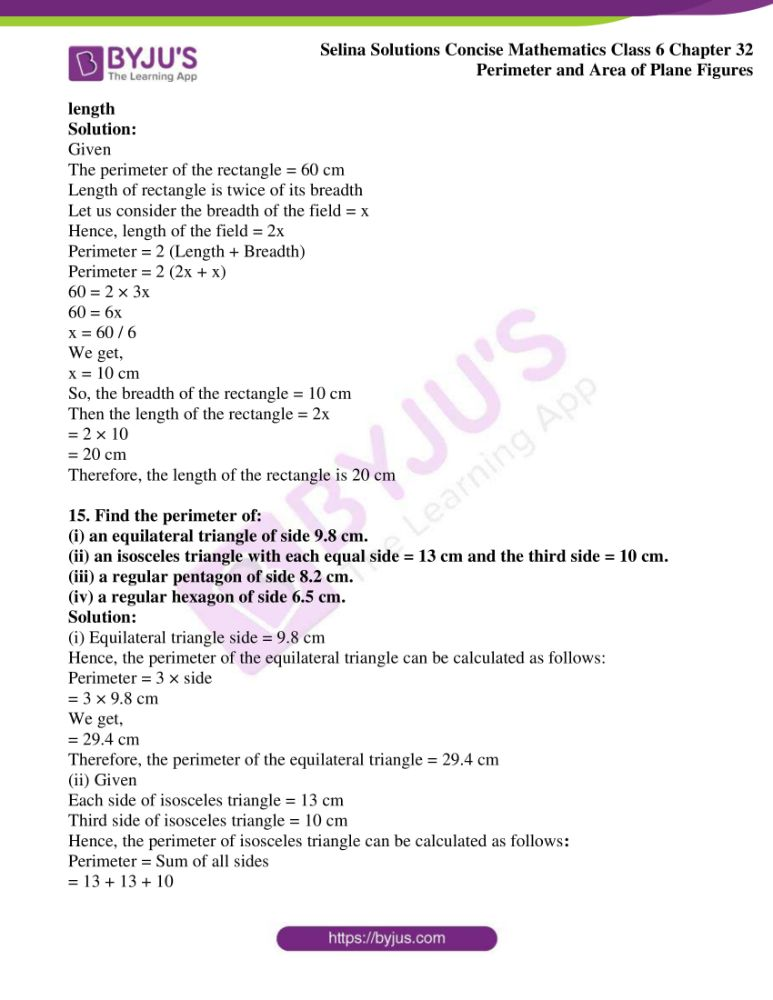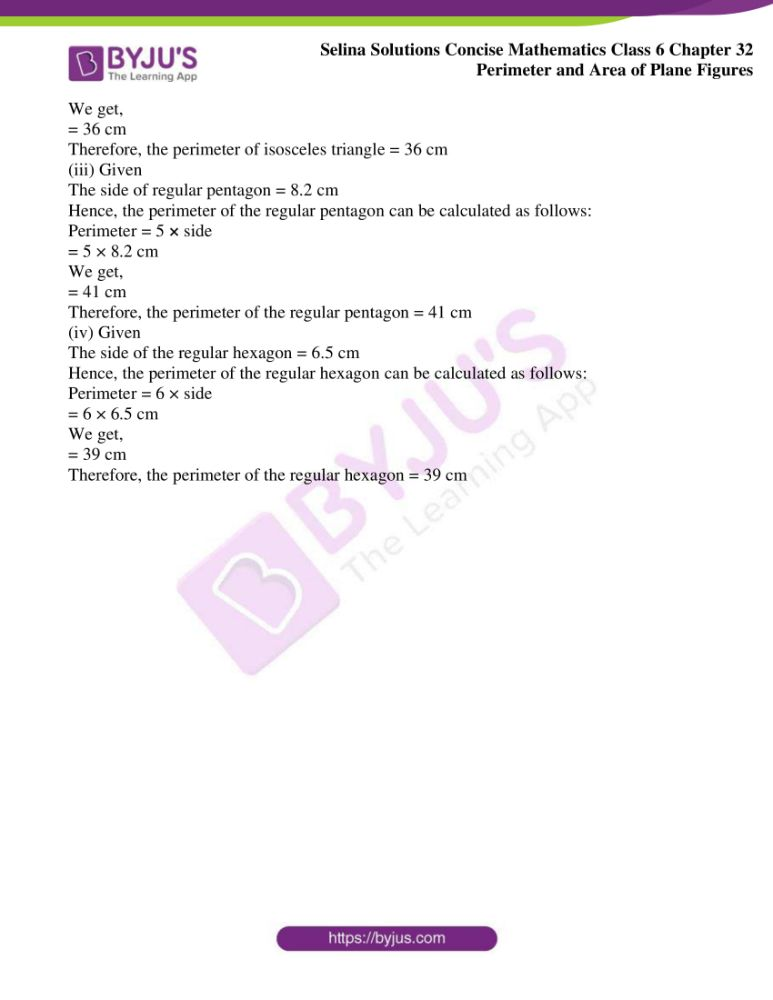### Access another exercise of Selina Solutions Concise Mathematics Class 6 Chapter 32 Perimeter and Area of Plane Figures

Exercise 32(B) Solutions

### Access Selina Solutions Concise Mathematics Class 6 Chapter 32 Perimeter and Area of Plane Figures Exercise 32(A)

Exercise 32(A)

1. What do you understand by a plane closed figure?

Solution:

Any geometrical plane figure which is bounded by straight or curved lines in a plane is called a plane closed figure

Following figures is a plane closed figure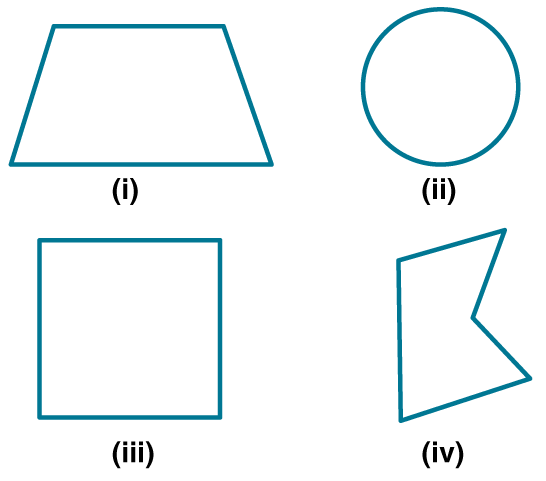2. The interior of a figure is called region of the figure. Is this statement true?

Solution:

The interior of the figure along with its boundary is called region of the figure. Hence, the given statement is true.

3. Find the perimeter of each of the following closed figures:

Solution:

The perimeter of the closed figures can be calculated as follows

Perimeter = Sum of all the sides

Perimeter = AB + AC + CD + DG + GH + FH + EF + BE

= 15 + 5 + 10 + 25 + 15 + 5 + 10 + 25

= 110 cm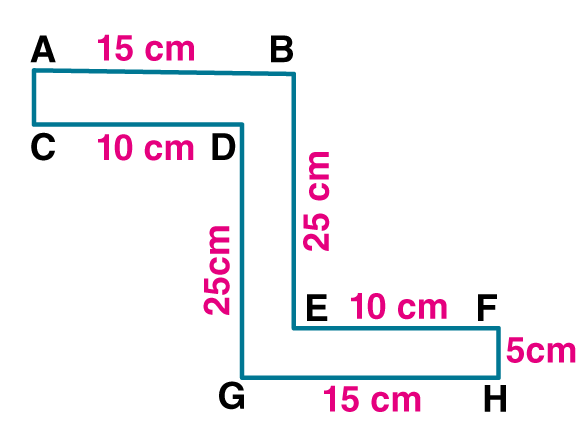Therefore, the perimeter of the closed figure = 110 cm

(ii) The perimeter of the closed figures can be calculated as follows

Perimeter = Sum of all the sides

Perimeter = AB + AC + CD + DG + BF + EF + EH + GH

= 20 + 4 + 8 + 20 + 4 + 8 + 20 + 4

= 88 cm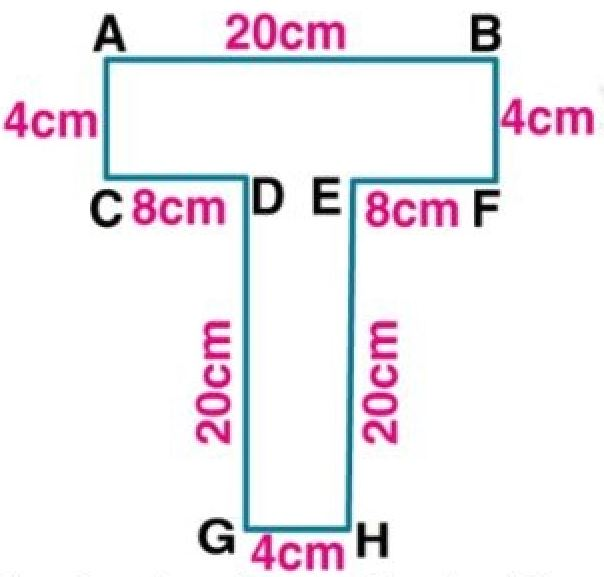Therefore, the perimeter of the closed figure = 88 cm

4. Find the perimeter of a rectangle whose:

(i) length = 40 cm and breadth = 35 cm

(ii) length = 10 m and breadth = 8 m

(iii) length = 8 m and breadth = 80 cm

(iv) length = 3.6 m and breadth = 2.4 m

Solution:

(i) Given

Length of the rectangle = 40 cm

Breadth of the rectangle = 35 cm

Hence, the perimeter of the rectangle is calculated as follows

Perimeter = 2 (Length + Breadth)

Perimeter = 2 (40 cm + 35 cm)

= 2 × 75 cm

= 150 cm

= 150 / 100 m

We get,

= 1.5 m

Hence, the perimeter of the rectangle = 1.5 m

(ii) Given

Length of the rectangle = 10 m

Breadth of the rectangle = 8 m

Hence, the perimeter of the rectangle is calculated as follows:

Perimeter = 2 (Length + Breadth)

Perimeter = 2 (10 m + 8 m)

= 2 × 18 m

We get,

= 36 m

Hence, the perimeter of the rectangle = 36 m

(iii) Given

Length of the rectangle = 8 m

Breadth of the rectangle = 80 cm

We know that,

100 cm = 1 metre

Hence, the breadth can be converted into metre from centimetre as below

= 80 / 100 m

We get,

= 0.8 m

Hence, the perimeter of the rectangle is calculated as follows:

Perimeter = 2 (Length + Breadth)

Perimeter = 2 (8 m + 0.8 m)

= 2 × 8.8 m

We get,

= 17.6 m

Hence, the perimeter of the rectangle = 17.6 m

(iv) Given

Length of the rectangle = 3.6 m

Breadth of the rectangle = 2.4 m

Hence, the perimeter of the rectangle is calculated as follows:

Perimeter = 2 (Length + Breadth)

Perimeter = 2 (3.6 m + 2.4 m)

= 2 × 6 m

We get,

= 12 m

Hence, the perimeter of the rectangle = 12 m

5. If P denotes perimeter of a rectangle, l denotes its length and b denotes its breadth, find:

(i) l, if P = 38 cm and b = 7 cm

(ii) b, if P = 3.2 m and l = 100 cm

(iii) P, if l = 2 m and b = 75 cm

Solution:

(i) Given

P = 38 cm and b = 7 cm

Length of the rectangle (l) can be calculated as below

Length (l) = P / 2 – b

= 38 / 2 – 7 cm

We get,

= 19 cm – 7 cm

= 12 cm

Hence, the length of the rectangle = 12 cm

(ii) Given

P = 3.2 m and l = 100 cm

The breadth of the rectangle (b) can be calculated as follows

Here, the length is in centimetre. Hence, it can be converted into metre from centimetre as below

Length = 100 cm

= 100 / 100 m

Breadth (b) = P / 2 – 1

= 3.2 / 2 – 1 m

= 1.6 m – 1 m

We get,

= 0.6 m

Hence, the breadth (b) of the rectangle = 0.6 m

(iii) Given

L = 2 m and b = 75 cm

The perimeter of the rectangle can be calculated as below:

We know that,

100 cm = 1 m

Here, the breadth is in centimetre. Hence, it can be converted into metre from centimetre as below

= 75 / 100 m

We get,

= 0.75 m

Perimeter = 2 (Length + Breadth)

Perimeter = 2 (2 m + 0.75 m)

= 2 × 2.75 m

We get,

= 5.5 m

Hence, the perimeter of the rectangle = 5.5 m

6. Find the perimeter of a square whose each side is 1.6 m.

Solution:

Given

Each side of a square = 1.6 m

Hence, the perimeter of a square can be calculated as follow:

Perimeter = 4 × side

= 4 × 1.6 m

We get,

= 6.4 m

Hence, the perimeter of a square = 6.4 m

7. Find the side of the square whose perimeter is 5 m.

Solution:

Given

The perimeter of the square = 5 m

Hence, the side of the square can be calculated as below

side = Perimeter / 4

= (5 / 4) m

We get,

= 1.25 m

Hence, the side of the square = 1.25 m

8. A square field has each side 70 m whereas a rectangular field has length = 50 m and breadth = 40 m. Which of the two fields has greater perimeter and by how much?

Solution:

Given

The side of square field = 70 m

Length of a rectangular field = 50 m

Breadth of a rectangular field = 40 m

So,

The Perimeter of the square field = 4 × side

= 4 × 70 m

We get,

= 280 m

The Perimeter of the rectangular field = 2 (length + breadth)

= 2 (50 m + 40 m)

= 2 × 90 m

We get,

= 180 m

Therefore, the perimeter of the square field is greater than the perimeter of rectangular field by 280 m – 180 m = 100 m

9. A rectangular field has length = 160 m and breadth = 120 m. Find:

(i) the perimeter of the field

(ii) the length of fence required to enclose the field

(iii) the cost of fencing the field at the rate of 80 per metre

Solution:

(i) Given

Length of the rectangular field = 160 m

Breadth of the rectangular field = 120 m

Hence, the perimeter of the rectangular field can be calculated as below:

Perimeter = 2 (Length + Breadth)

Perimeter = = 2 (160 m + 120 m)

= 2 × 280 m

We get,

= 560 m

Therefore, the perimeter of the field = 560 m

(ii) The length of fence required to enclose the field is equal to the perimeter of the rectangular field. Therefore, the length of the fence is 560 m

(iii) Given

The cost of fencing the field per metre = Rs 80

Hence, total cost of fencing the field can be calculated as follows:

Total Cost = Length of fence × Rate of fence

= 560 m × Rs 80 per metre

We get,

= Rs 44, 800

Therefore, total cost of fencing the field = Rs 44,800

10. Each side of a square plot of land is 55 m. Find the cost of fencing the plot at the rate of Rs 32 per metre.

Solution:

Given

Each side of a square field = 55 m

Hence, the perimeter of square field can be calculated as follows:

Perimeter = 4 × side

= 4 × 55 m

We get,

= 220 m

We know that, the length of fence required to enclose the field is the perimeter of the square field.

Therefore, the length of fence = 220 m

Given

Cost of fence per metre = Rs 32

Hence, total cost of fencing the field can be calculated as follows:

Total cost of fencing the field = Length of fence × Rate of fence

= 220 m × 32

We get,

= Rs 7040

Therefore, total cost of fencing the field = Rs 7040

11. Each side of a square field is 70 cm. How much distance will a boy walk in order to make?

(i) one complete round of this field?

(ii) 8 complete rounds of this field?

Solution:

(i) Given

Each side of a square fields = 70 m

Distance covered to complete one round of the field by the boy is equal to the perimeter of the field

Hence, the perimeter of square field can be calculated as below

Perimeter = 4 × side

= 4 × 70 m

We get,

= 280 m

Therefore, the distance covered by the boy to complete one round of the field is 280 m

(ii) The distance covered to complete 8 rounds of the field can be calculated as follows:

Distance = 8 × Perimeter

= 8 × 280

We get,

= 2240 m

Therefore, the distance covered by the boy to complete 8 rounds of the field is 2240 m

12. A school playground is rectangular in shape with length = 120 m and breadth = 90 m. Some school boys run along the boundary of the play-ground and make 15 complete rounds in 45 minutes. How much distance they run during this period?

Solution:

Given

Length of rectangular playground = 120 m

Breadth of rectangular playground = 90 m

Hence, the perimeter of rectangular playground can be calculated as follows:

Perimeter = 2 (Length + Breadth)

Perimeter = 2 (120 m + 90 m)

= 2 × 210 m

We get,

= 420 m

Hence, distance covered by the boy in one round = 420 m

Therefore, total distance covered by the boy to complete 15 rounds can be calculated as follows:

Distance = 15 × Perimeter

= 15 × 420

We get,

= 6300 m

Therefore, distance covered by the boys to completer 15 rounds is 6300 m

13. Mohit makes 8 full rounds of a rectangular field with length = 120 m and breadth = 75 m. John makes 10 full rounds of a square field with each side 100 in. Find who covers larger distance and by how much?

Solution:

Given

Length of the rectangular field = 120 m

Breadth of the rectangular field = 75 m

Side of square field = 100 m

Distance covered by Mohit in one round is equal to the perimeter of the rectangular field

Hence, the perimeter of the rectangular field can be calculated as follows:

Perimeter = 2 (Length + Breadth)

= 2 × 195 m

We get,

= 390 m

Now, the total distance covered by the Mohit to complete the 8 rounds can be calculated as follows:

Distance = 8 × Perimeter

= 8 × 390

We get,

= 3120 m

Distance covered by John in one round is equal to the perimeter of the square field

Hence, the perimeter of square field can be calculated as follows:

Perimeter = 4 × side

= 4 × 100 m

We get,

= 400 m

Therefore the total distance covered by John to complete 10 rounds can be calculated as follows:

Distance = 10 × Perimeter

= 10 × 400 m

We get,

= 4000 m

Difference between the distance covered by Mohit and John is,

= 4000 m – 3120 m

We get,

= 880 m

Therefore, John covers larger distance by 880 m than the distance covered by Mohit.

14. The length of a rectangle is twice of its breadth. If its perimeter is 60 cm, find its length

Solution:

Given

The perimeter of the rectangle = 60 cm

Length of rectangle is twice of its breadth

Let us consider the breadth of the field = x

Hence, length of the field = 2x

Perimeter = 2 (Length + Breadth)

Perimeter = 2 (2x + x)

60 = 2 × 3x

60 = 6x

x = 60 / 6

We get,

x = 10 cm

So, the breadth of the rectangle = 10 cm

Then the length of the rectangle = 2x

= 2 × 10

= 20 cm

Therefore, the length of the rectangle is 20 cm

15. Find the perimeter of:

(i) an equilateral triangle of side 9.8 cm.

(ii) an isosceles triangle with each equal side = 13 cm and the third side = 10 cm.

(iii) a regular pentagon of side 8.2 cm.

(iv) a regular hexagon of side 6.5 cm.

Solution:

(i) Equilateral triangle side = 9.8 cm

Hence, the perimeter of the equilateral triangle can be calculated as follows:

Perimeter = 3 × side

= 3 × 9.8 cm

We get,

= 29.4 cm

Therefore, the perimeter of the equilateral triangle = 29.4 cm

(ii) Given

Each side of isosceles triangle = 13 cm

Third side of isosceles triangle = 10 cm

Hence, the perimeter of isosceles triangle can be calculated as follows:

Perimeter = Sum of all sides

= 13 + 13 + 10

We get,

= 36 cm

Therefore, the perimeter of isosceles triangle = 36 cm

(iii) Given

The side of regular pentagon = 8.2 cm

Hence, the perimeter of the regular pentagon can be calculated as follows:

Perimeter = 5 × side

= 5 × 8.2 cm

We get,

= 41 cm

Therefore, the perimeter of the regular pentagon = 41 cm

(iv) Given

The side of the regular hexagon = 6.5 cm

Hence, the perimeter of the regular hexagon can be calculated as follows:

Perimeter = 6 × side

= 6 × 6.5 cm

We get,

= 39 cm

Therefore, the perimeter of the regular hexagon = 39 cm